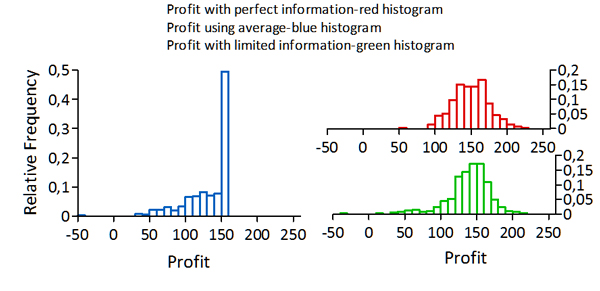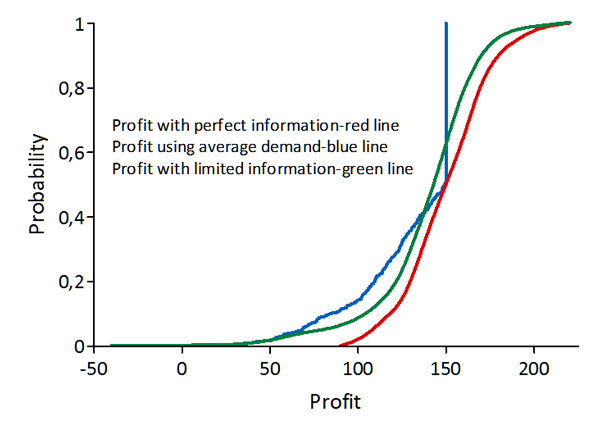# The Value of Information

This entry is part 4 of 4 in the series A short presentation of S@R

Enterprise risk management (ERM) only has value to those who know that the future is uncertain

First, they need to have a product or service that people will buy. They need revenues.

Second, they need to have the ability to provide that product or service at a cost less than what their customers will pay. They need profits. Once they have revenues and profits, their business is a valuable asset.

So third, they need to have a system to avoid losing that asset because of unforeseen adverse experience. They need risk management.

The top CFO concern is the firm’s ability to forecast results and the first stepping-stone in the process of forecasting results is to forecast demand – and this is where ERM starts.

The main risk any firm faces is the variability (uncertainty) of demand. Since all production activities like procurement of raw materials, sizing of work force, investment in machinery etc. is based on expected demand the task of forecasting future demand is crucial. It is of course difficult and in most cases not possible to perfectly forecast demand, but it is always possible to make forecasts that give better results than mere educated guesses.

We will attempt in the following to show the value of making good forecasts by estimating the daily probability distribution for demand. We will do this using a very simple model, assuming that:

1. Daily demand is normal distributed with expected sales of 100 units and a standard deviation of 12 units,
2. the product can not be stocked,
3. it sells at \$4 pr unit, has a variable production cost of \$2 and a fixed production cost of \$50.

Now we need to forecast the daily sales. If we had perfect information about the demand, we would have a probability distribution for daily profit as given by the red histogram and line in the graphs below.

• One form of forecast (average) is the educated guess using the average daily sales (blue histogram). As we can see from the graphs, this forecast method gives a large downside (too high production) and no upside (too low production).
• A better method (limited information) would have been to forecast demand by its relation to some other observable variable. Let us assume that we have a forecast method that gives us a near perfect forecast in 50% of the cases and a probability distribution for the rest that is normal distributed with expected sales as for demand, but with a standard deviation of six units (green histogram).With the knowledge we have from (selecting strategy) we clearly se that the last forecast strategy is stochastic dominant to the use of average demand as forecast.So, what is the value to the company of more informed forecasts than the mere use of expected sales? The graph below gives the distribution for the differences in profit (percentage) using the two methods. Over time, the second method  will give on average an 8% higher profit than just using the average demand as forecast.However, there is still another seven to eight percent room for further improvement in the forecasting procedure.

If the company could be reasonable sure of the existence of a better forecast model than using the average, it would be a good strategy to put money into a betterment. In fact it could use up to 8% of all future profit if it knew that a method as good as or better than our second method existed.

Series Navigation<< Risk, price and value